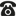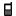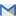## Thống kê

• truy cập   (chi tiết)
trong hôm nay
• lượt xem
trong hôm nay
• thành viên
• ## Tin tức thư viện

#### Khắc phục hiện tượng không xuất hiện menu Bộ công cụ Violet trên PowerPoint và WordKính chào các thầy, cô. Khi cài đặt phần mềm , trên PowerPoint và Word sẽ mặc định xuất hiện menu Bộ công cụ Violet để thầy, cô có thể sử dụng các tính năng đặc biệt của phần mềm ngay trên PowerPoint và Word. Tuy nhiên sau khi cài đặt phần mềm , với nhiều máy tính sẽ...
Xem tiếp

## Hướng dẫn sử dụng thư viện

#### Bài 4: Quản lí ngân hàng câu hỏi và sinh đề có điều kiệnỞ , , chúng ta đã biết cách tạo một đề thi từ ngân hàng có sẵn hay tự nhập câu hỏi, tạo cây thư mục để chứa đề thi cho từng môn. Trong bài này chung ta tiếp tục tìm hiểu cách xây dựng và quản lý ngân hàng câu hỏi mà mình đã đưa lên và...
Xem tiếp

## Liên hệ quảng cáo

•(024) 66 745 632
•096 181 2005
•contact@bachkim.vn

## Một số đề và bài giải Toán OLYMPIAD bậc Tiểu học• 0 / 0
•Nhấn vào đây để tải về
Báo tài liệu có sai sót
Nhắn tin cho tác giả
(Tài liệu chưa được thẩm định)
Nguồn:
Người gửi: Tôn Nữ Bích Vân (trang riêng)
Ngày gửi: 23h:42' 07-03-2009
Dung lượng: 50.5 KB
Số lượt tải: 68
Số lượt thích: 0 người


Giới thiệu cùng các em một vài bài toán trích từ các đề thi Olympiad bậc tiểu học kèm theo bài giải bằng tiếng Anh để các em tham khảo.
TÔN NỮ BÍCH VÂN

( Suppose today is Tuesday. What day of the week will it be 100 days from now?
( I have four 30¢ stamps and three 50¢ stamps. Using one or more of these stamps, how many different amounts of postage can I make?
( Find the sum of the counting numbers from 1 to 25 inclusive. In other words, if S = 1 + 2 + 3 + ... + 24 + 25, find the value of S.
( In a stationery store, pencils have one price and pens have another price. 2 pencils and 3 pens cost 90¢. But 3 pencils and 2 pens cost 85¢. How much does 1 pencil cost?
( A work crew of 3 people requires 3 weeks and 2 days to do a certain job. How long would it take a work crew of 4 people to do the same job if each person of both crews works at the same rate as each of the others?
Note: Each week contains 6 work days

SOLUTIONS

( Every 7 days from "today" will be Tuesday. Since 98 is a multiple of 7, the 98th day from today will be Tuesday. Then the 100th day from today will be Thursday.

( Method 1
List the amounts in an organised manner.

Amounts
Number

Amounts from 30¢ stamps:
30, 60, 90, 120
4

Amounts from 50¢ stamps:
50, 100, 150
3

Amounts from combining
30¢ stamps and 50¢ stamps:

30+50, 30+100, 30+150

3


60+50, 60+100, 60+150
3


90+50, 90+100, 90+150
3


120+50, 120+100, 120+150
3


Total
19



Method 2
The number of choices we have in using the 30¢ stamps is 5; we can use either 0, 1, 2, 3 or 4 of the 30¢ stamps. Similarly, we have 4 choices with respect to the 50¢ stamps; we can use either 0, 1, 2 or 3 of the 50¢ stamps. Each of the 5 choices for stamps. This gives a total of 20¢ combinations. However, this total includes the combination of zero 30¢ stamps and zero 50¢ stamps. Since one or more of the stamps must be used, we exclude the combination of none of each. Therefore, 19 different amounts of postage can be made.

( Method 1:
Arrange the numbers like this: 1 + 25 = 25
2 + 23 = 25
3 + 22 = 25

12 + 13 = 25
25 = 25
Method 2:
Given
(1)
 S = 1 + 2 + 3 + ... + 23 + 24 + 25

Reverse order of right side of (1)
(2)
 S = 25 + 24 + 23 + ... + 3 + 2 + 1

Add (1) and (2)
(3)
2S = 26 + 26 + 26 + ... + 26 + 26 + 26

Simplify the right side of (3)
(4)
2S = 26 x 25

Divide both sides of (4) by 2
(5)
 S = 13 x 25 or 325

The required sum is 325




( Method 1:
By combining both purchases, we find that 5 pencils and 5 pens cost 175¢. Then 1 pencil and 1 pen cost 35¢, or 2 pencils and 2 pens cost 70¢. Since 3 pencils and 2 pens cost 85¢, 1 pencil costs 15¢.
Method 2:
Algebra: Let x represent the cost of one pencil and y the cost of one pen.
Give
(1)
2x + 3y = 90

Give
(2)
3x + 2y = 85

Multiply both sides of (2) by 3
(3)
9x + 6y = 255

Multiply both sides of (1) by 2
(4)
4x + 6y = 180

Subtract (4) from (3)
(5)
5x = 75

Divide both sides of (5) by 5
(6)
 x = 15

Answer: A pencil costs 15¢




( Each person of the work crew of 3 people worked 20 days. Thus the number of individual work days needed to

Gửi ý kiến